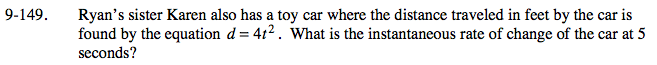### Home > PC > Chapter 9 > Lesson 9.3.4 > Problem9-149

9-149.

Ryan’s sister Karen also has a toy car where the distance traveled in feet by the car is found by the equation d = 4t2. What is the instantaneous rate of change of the car at 5 seconds? Homework Help ✎Find the slope function and substitute t = 5:

$\lim_{ h \to 0 }\frac{f(t+h)-f(t)}{h}$

IROC = 40 feet/second